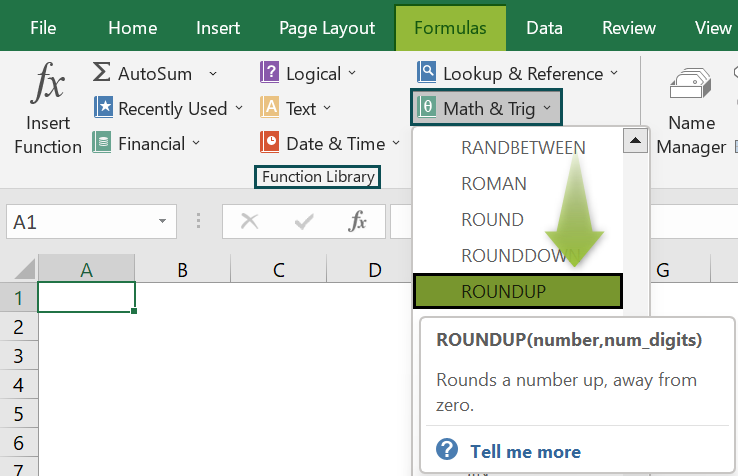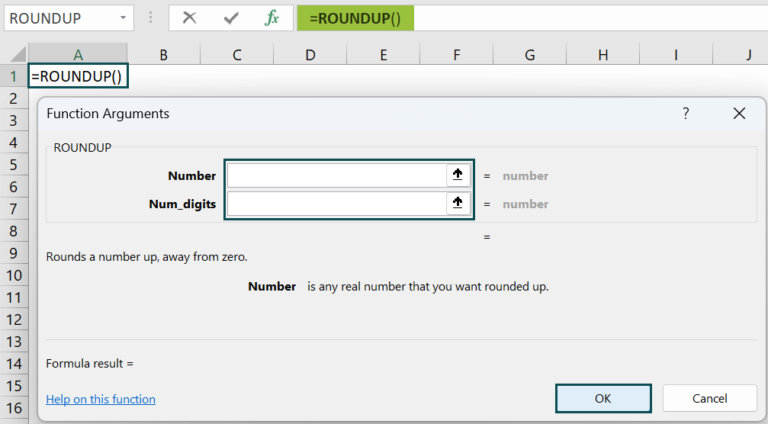# ROUNDUP Formula in ExcelArticle byExcelMojo Team## What Is ROUNDUP Formula In Excel?

The ROUNDUP formula in Excel is used for rounding up the number after the decimal. The function is categorized under the Math & Trig function of the Function Library.

The ROUNDUP Function in Excel allows users to choose how many digits, numbers could be rounded up; we can either retain any number of digits after the decimal or completely round off the selected numbers. We can also use positive or negative signs with num_digit to move the decimal right or left side.

For example, enter the number 1.2345 as the number argument and 2 as the num_digits argument to round up the number. Enter the formula =ROUND(1.2345,2) in cell A1. The result displayed will be 1.24.

Likewise, we can use roundup formula in excel.

###### Key Takeaways
• The ROUNDUP Function in Excel rounds the numbers upto 1-9.
• The ROUNDUP function can round either to the left or right of the decimal point.
• If the value of the “num_digits” argument is 0, then a number is rounded to the nearest whole number.
• Incase, “num_digits” argument is greater than 0; a number is rounded to the right of the decimal point.
• The value of the “num_digits” argument is less than 0; a number is rounded to the left of the decimal point.

### ROUNDUP() Excel Formula

The syntax of the ROUNDUP Excel Function is

• number = This is the numerical value we want to round up.
• num_digits = This is the number of digits we want to round up.

Unlike other functions, both the arguments in the ROUNDUP Excel function are mandatory.

### How To Use ROUNDUP Excel Function?

#### #1 – Access From The Excel Ribbon

• Choose the empty cell which will contain the result.
• Go to the Formulas tab and click it.
• Select the Math & Trig option from the drop-down menu.
• Select ROUNDUP from the drop-down menu.
• A window called Function Arguments appears.
• As the number of arguments, enter the value in the number & num_digits.
• Select OK.

#### #2 – Enter The Worksheet Manually

1. Select an empty cell for the output.
2. Type =ROUNDUP( in the selected cell. Alternatively, type =R and double-click the ROUNDUP function from the list of suggestions shown by Excel.
3. Press the Enter key.

The succeeding example rounds up the values, and we will calculate the rounded value using Excel ROUNDUP formula.

In the table,

• Column A contains the Number.
• Column B contains the Output.

The steps to round the value by the ROUND formula in Excel are as follows:

1. To begin with, we should select the cell where we will enter the formula and calculate the result. The selected cell, in this case, is cell B2.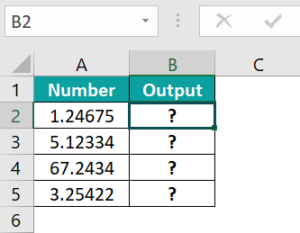2. Next, we will enter the ROUNDUP formula in cell B2.

3. Then, enter the value of ‘number’ as A2, i.e., the number value.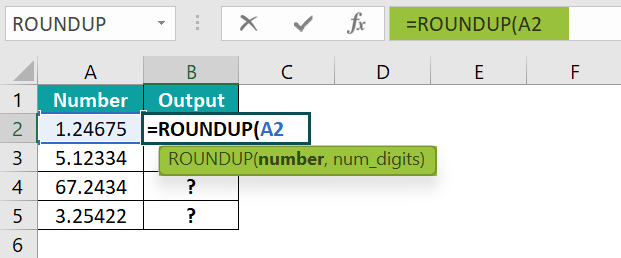4. Now, enter the value of num_digits as 3, i.e., the number of digits after the decimal.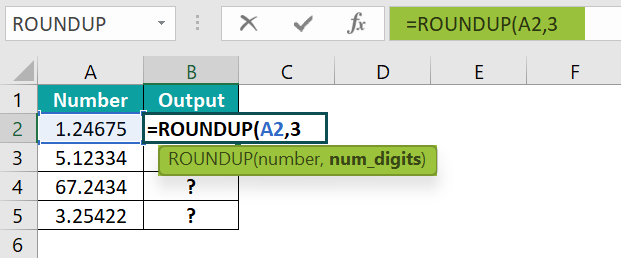5. Next, the complete formula is =ROUNDUP(A2,3) in cell B2.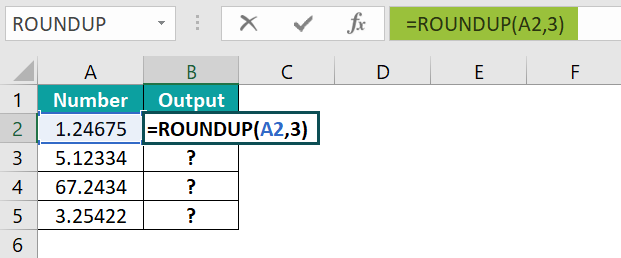6. After entering each value in the preceding step, press the Enter key. The results in cell B2 is returned as 1.247.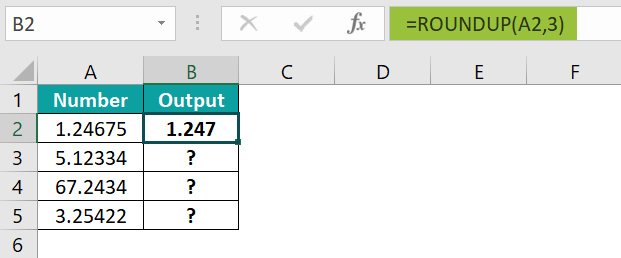7. Next, press Enter and drag the cursor to cell B5, as shown in the following image.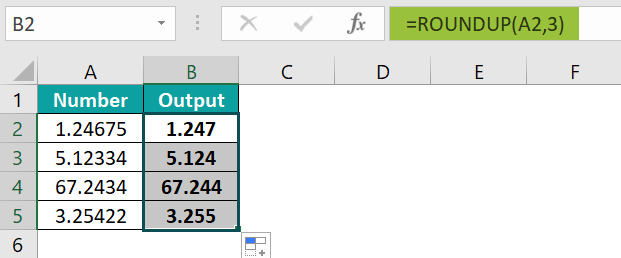Similarly, we can use roundup function in excel to obtain the results.

### Examples

#### Example #1

The following example rounds up the values, and the num_digits value will be less than zero. We will calculate the rounded value using ROUNDUP function in excel.

In the table,

• Column A contains the Number.
• Column B contains the Num_digits.
• Column C contains the Output.

The steps to round the value by the ROUND Function in Excel are as follows:

• Step 1: First, select the cell where we will enter the formula and calculate the result. The selected cell, in this case, is cell C2.
• Step 2: Next, we will enter the ROUNDUP formula in cell C2.
• Step 3: Then, enter the value of number as A2, i.e., the number value.
• Step 4: Now, enter the value of num_digits as B2, i.e., the number of digits after the decimal; in this case, the num_digits value is negative.
• Step 5: So, the complete formula is =ROUNDUP(A2,B2) in cell C2.
• Step 6: After entering each value in the preceding step, press the Enter key. The results in cell C2 are as 30 in the image below.
• Step 7: Next, press Enter and drag the cursor to cell C5, as shown in the following image.

Likewise, we can use roundup formula in excel.

#### Example #2

The succeeding example rounds up the values, and the num_digits value will equal zero. We will calculate the rounded value using Excel ROUNDUP function.

In the table,

• Column A contains the Number.
• Column B contains the Num_digits.
• Column C contains the Output.

The steps to round the value by the ROUND Function in Excel are as follows:

• Step 1: To begin with, select the cell where we will enter the formula and calculate the result. The selected cell, in this case, is cell C2.
• Step 2: Next, enter the ROUNDUP formula in cell C2.
• Step 3: Now, enter the value of number as A2, i.e., the number value.
• Step 4: Next, enter the value of num_digits as B2, i.e., the number of digits after the decimal; in this case, the num_digits value is equal to zero.
• Step 5: So, the complete formula is =ROUNDUP(A2,B2) in cell C2.
• Step 6: After entering each value in the preceding step, press the Enter key. The results in cell C2 is returned as 10 in the image below.
• Step 7: Next, press Enter and drag the cursor to cell C5, as shown in the following image.

Similarly, we can use roundup function in excel to obtain the results.

#### Example #3

The succeeding example rounds up the values, and the num_digits value will be greater than zero. We will calculate the rounded value using the Excel ROUNDUP function.

In the table,

• Column A contains the Number.
• Column B contains the Num_digits.
• Column C contains the Output.

The steps to round the value by the ROUND Function in Excel are as follows:

• Step 1: First, select the cell where we will enter the formula and calculate the result. The selected cell, in this case, is cell C2.
• Step 2: Next, we will enter the ROUNDUP formula in cell C2.
• Step 3: Then, enter the value of number as A2, i.e., the number value.
• Step 4: Now, enter the value of num_digits as B2, i.e., the number of digits after the decimal; in this case, the num_digits value is greater than zero.
• Step 5: So, the complete formula is =ROUNDUP(A2,B2) in cell C2.
• Step 6: After entering each value in the preceding step, press the Enter key. The results in cell C2 is returned as 21.4 in the image below.
• Step 7: Next, press Enter and drag the cursor to cell C5, as shown in the following image.

Likewise, using ROUNDUP function in excel, we can obtain the results.

### Important Things To Note

• The ROUNDUP formula in Excel for Office 365, Excel 2019, Excel 2016, Excel 2013, Excel 2011 for Mac, Excel 2010, Excel 2007, Excel 2003, Excel XP, Excel 2000
• The ROUNDUP function returns a numeric value.

1. What does the ROUNDUP function do in Excel?

The ROUNDUP function rounds up a number to a given number of decimal places. The function rounds a number up, away from zero. It is a built-in function in excel, and it falls under the Math & Trig category function.

The ROUNDUP function is part of the ROUND function family. The ROUND function can round to the right or left of the decimal point, and the ROUNDUP function always rounds numbers 1-9 up.

The syntax of the ROUNDUP formula is shown below;
=ROUNDUP(number,num_digits)

2. How does the ROUNDUP function work in Excel?

The ROUNDUP function works in Excel by following the below steps;
First, select an empty cell for the output.
Next, type =ROUNDUP( in the selected cell. Alternatively, type =R and double-click the ROUNDUP function from the list of suggestions shown by Excel.
Finally, press the Enter key.

For example, we will round up the values and calculate the rounded value using the Excel ROUNDUP formula.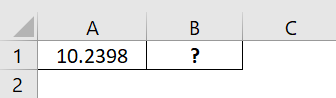The steps to round the value by the ROUND Function in Excel are as follows:

• Step 1: To begin with, select the cell where we will enter the formula and calculate the result. The selected cell, in this case, is cell B1.

• Step 2: Next, we will enter the ROUNDUP formula in cell B1.

• Step 3: Then, enter the value of number as A1, i.e., the number value.

• Step 4: Now, enter the value of num_digits as 3, i.e., the number of digits after the decimal.

• Step 5: So, the complete formula is =ROUNDUP(A1,3) in cell B1.

• Step 6: Finally, press the Enter key. The result is obtained in cell B1 as 10.24.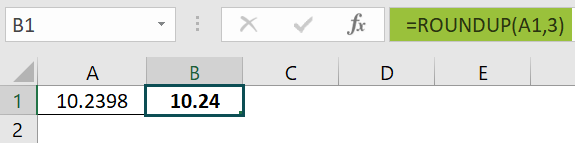Similarly, we can use roundup function in excel.

3. Where is the ROUNDUP function in Excel?

We can activate the ROUNDUP Function in Excel using the following steps:
Choose the empty cell which will contain the result.
Go to the Formulas tab and click it.
Select the Math & Trig option from the drop-down menu.
Select ROUNDUP from the drop-down menu.
A window called Function Arguments appears.
As the number of arguments, enter the value in the number & num_digits.
Select OK.